# 【高精度】 利用分段存储的方法储存大数与运算

=====================================================================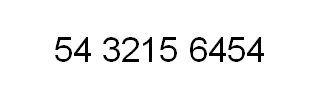int first, seconed, thrid;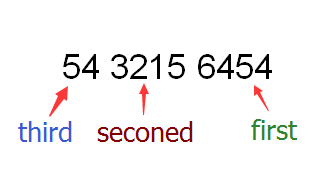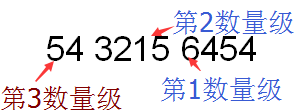--------------------------------------------

#define SCALE ScaleNum  //数量级之间的进制，一般设成10的幂次方数

struct BigNumber{
int num[MAX]; //最高能存储MAX个数量级位数的数
int oom; //该数的最高段存储位置，可以看成是该数的数据规模

};

--------------------------------------------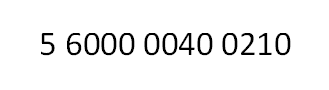#include <iostream>
#define SCALE 10000
struct BigNumber{
int num;
int oom;
};

int Bits(int x) //计算一个数有几位，时间复杂度为O(lg ScaleNum)
{
if(x == 0) return 1;

int count = 1;
for(int i=10; i<=SCALE ;i*=10){
if(x/i == 0) return count;
count++;
}
}

void Print(BigNumber BigNum, int PrintBits = 4) //输出大数BigNum，因为4位为1段，故每段应该打印出PrintBits = 4位数字
{
cout<<BigNum.num[BigNum.oom];//最高位可以直接输出

int zero_fill; //计算在前面需要补几个0的变量
for(int i=BigNum.oom-1; i>=0 ;i--){ //大循环，每次循环输出一个段
zero_fill = PrintBits - Bits(BigNum.num[i]);//补0数量 = 应该打印的位数 - 当前段的数的位数

for(int count=0; count<zero_fill ;count++)//补0小循环
cout<<0;

cout<<BigNum.num[i];
}
cout<<endl;
}

=====================================================================

-----------------------------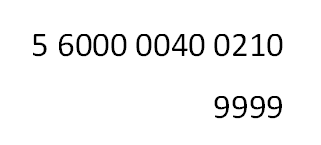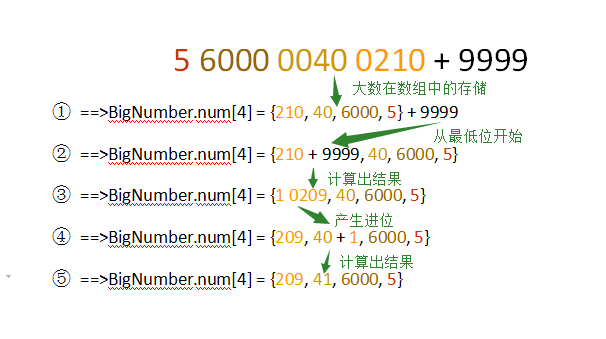#include <iostream>
#define SCALE 10000
BigNumber operator+ (int x)const
{
BigNumber R = *this;

int temp = x;

int pos = 0;
while(temp){
R.num[pos] += temp;
temp = R.num[pos] / SCALE;
R.num[pos++] %= SCALE;
}

/*数量级扩增判断*/
if(pos > R.oom && R.num[pos]) R.oom = pos;

return R;
};

---------------------------------------------------

---

5 6000 0040 0210 * 9999做例子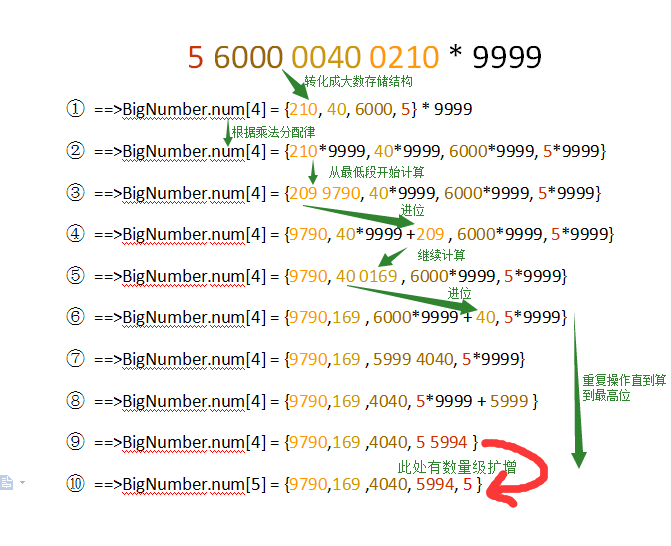BigNumber operator* (int x) const
{
BigNumber R = *this;
int last_carry = 0; //从前一位取得的进位
int now_carry; //当前位取得的进位
/*计算*/
for(int i=0; i<=R.oom ;i++){
now_carry = (R.num[i]*x+last_carry) / SCALE; //获得进位
R.num[i] = (R.num[i]*x+last_carry) % SCALE;

last_carry = now_carry;
}

/*数量级扩增判断*/
if(last_carry > 0){ //若最后的进位不为0，则扩增数量级
R.oom++;
R.num[R.oom] = last_carry;
}

return R;
};

=====================================================================

=====================================================================

#include <iostream>
#include <cstring>
#define SCALE 10000
using namespace std;

struct BigNumber{
int num;
int oom;

BigNumber operator+ (int x)const
{
BigNumber R = *this;

int temp = x;

int pos = 0;
while(temp){
R.num[pos] += temp;
temp = R.num[pos] / SCALE;
R.num[pos++] %= SCALE;
}

/*数量级扩增判断*/
if(pos > R.oom && R.num[pos]) R.oom = pos;

return R;
};

BigNumber operator* (int x) const
{
BigNumber R = *this;
int last_carry = 0; //从前一位取得的进位
int now_carry; //当前位取得的进位
/*计算*/
for(int i=0; i<=R.oom ;i++){
now_carry = (R.num[i]*x+last_carry) / SCALE; //获得进位
R.num[i] = (R.num[i]*x+last_carry) % SCALE;

last_carry = now_carry;
}

/*数量级扩增判断*/
if(last_carry > 0){ //若最后的进位不为0，则扩增数量级
R.oom++;
R.num[R.oom] = last_carry;
}

return R;
};
};

int Bits(int x) //计算一个数有几位，时间复杂度为O(lgScaleNum)
{
if(x == 0) return 1;

int count = 1;
for(int i=10; i<=SCALE ;i*=10){
if(x/i == 0) return count;
count++;
}
}

void Print(BigNumber BigNum, int PrintBits = 4) //输出大数BigNum，因为4位为1段，故每段应该打印出PrintBits = 4位数字
{
cout<<BigNum.num[BigNum.oom];//最高位可以直接输出

int zero_fill; //计算在前面需要补几个0的变量
for(int i=BigNum.oom-1; i>=0 ;i--){ //大循环，每次循环输出一个段
zero_fill = PrintBits - Bits(BigNum.num[i]);//补0数量 = 应该打印的位数 - 当前段的数的位数

for(int count=0; count<zero_fill ;count++)//补0小循环
cout<<0;

cout<<BigNum.num[i];
}
cout<<endl;
}

int main()
{
BigNumber big_num;

big_num.oom = 0;
memset(big_num.num, 0, sizeof(big_num.num));

/*算n的阶乘*/
int n;
cin>>n;
big_num.num = 1;
for(int i=n; i>=1; i--)
big_num = big_num * i;
Print(big_num);
/*之后加上9999*/
big_num = big_num + 9999;
Print(big_num);

return 0;
} 

09-044622

#### python中将list分段并保存为array类型

12-146089

#### 【操作系统】分段存储管理方式07-264458

#### python文件分段的两种方式

01-076888

#### 存储器分段和地址计算

05-17147

#### 利用固定长度分段存储大整数，并在系数表达式下实现四则运算（C语言实现）

05-1084

#### 判断集合数据是否连续，并将连续的部分分段存储

01-05502

05-21

#### 高精度数据存储问题

12-12

01-30240

#### 高精度计算——数据的接受方法和存储方法

11-302388

#### 存储器管理——分页、分段存储方式

11-253143

#### 基本分段存储管理方式

07-181万+

#### Python如何将列表分成均匀大小的块？

01-117178

#### 存储器地址的分段表示及物理地址的计算

11-281885

#### 使用 C# 分段读写 SQL Server 中 varbinary 字段中的数据

12-06

11-221183

#### C# 大规模数据分批与数据库交互

10-101749

03-11

#### HoloLens2开发入门教程

05-01©️2020 CSDN 皮肤主题: 游动-白 设计师: 上身试试点击重新获取扫码支付1.余额是钱包充值的虚拟货币，按照1:1的比例进行支付金额的抵扣。
2.余额无法直接购买下载，可以购买VIP、C币套餐、付费专栏及课程。余额充值